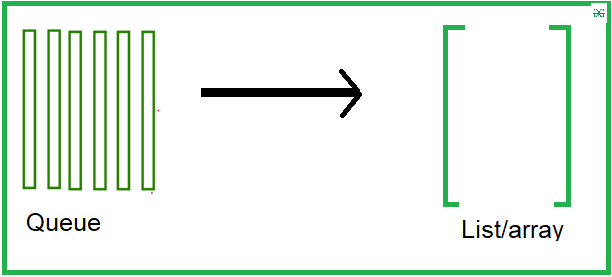Open in App
Not now

# Dumping queue into list or array in Python

• Last Updated : 31 Dec, 2020

Prerequisite: Queue in Python

Here given a queue and our task is to dump the queue into list or array. We are to see two methods to achieve the objective of our solution.Example 1:

In this example, we will create a queue using the collection package and then cast it into the list

## Python3

 `# Python program to ` `# demonstrate queue implementation ` `# using collections.dequeue ` `  `  `from` `collections ``import` `deque ` `  `  `# Initializing a queue ` `q ``=` `deque() ` `  `  `# Adding elements to a queue ` `q.append(``'a'``) ` `q.append(``'b'``) ` `q.append(``'c'``) ` ` `  `# display the queue ` `print``(``"Initial queue"``) ` `print``(q,``"\n"``) ` ` `  `# display the type ` `print``(``type``(q)) `

Output:

```Initial queue
deque(['a', 'b', 'c'])

<class 'collections.deque'>```

Let’s create a list and cast into it:

## Python3

 `# convert into list ` `li ``=` `list``(q) ` ` `  `# display ` `print``(``"Convert into the list"``) ` `print``(li) ` `print``(``type``(li)) `

Output:

```Convert into the list
['a', 'b', 'c']
<class 'list'>```

Example 2:

In this example, we will create a queue using the queue module and then cast it into the list.

## Python3

 `from` `queue ``import` `Queue ` ` `  `# Initializing a queue ` `que ``=` `Queue() ` ` `  `# Adding elements to a queue ` `que.put(``1``) ` `que.put(``2``) ` `que.put(``3``) ` `que.put(``4``) ` `que.put(``5``) ` ` `  `# display the queue ` `print``(``"Initial queue"``) ` `print``(que.queue) ` ` `  `# casting into the list ` `li ``=` `list``(que.queue) ` `print``(``"\nConverted into the list"``) ` `print``(li)`

Output:

```Initial queue
deque([1, 2, 3, 4, 5])

Converted into the list
[1, 2, 3, 4, 5]```

My Personal Notes arrow_drop_up
Related Articles